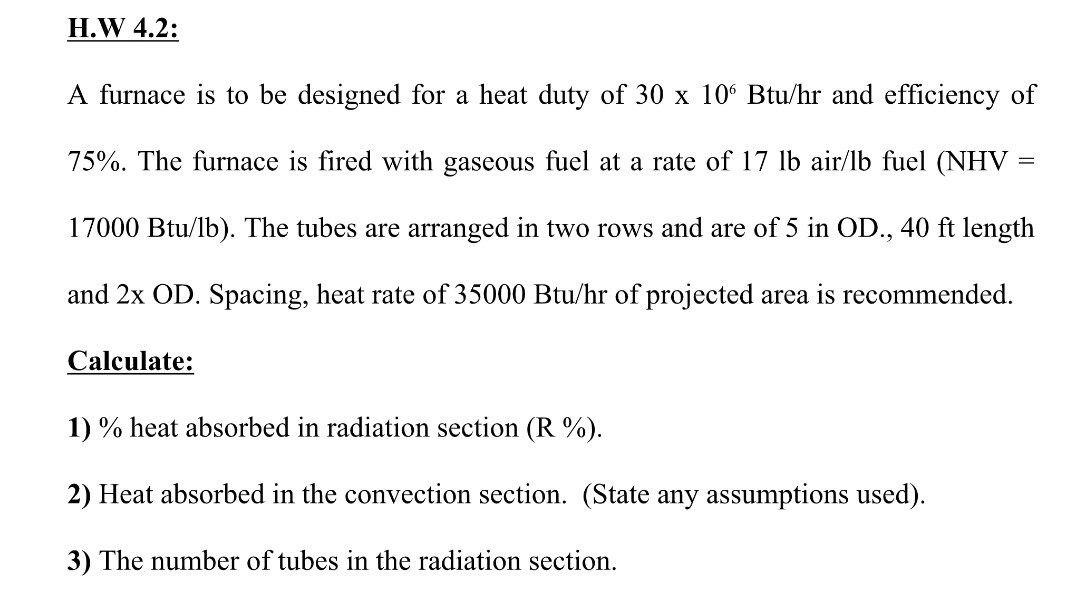# Question H.W 4.2: A furnace is to be designed for a heat duty of 30 x 106 Btu/hr and efficiency of 75%. The furnace is fired with gaseous fuel at a rate of 17 lb air/lb fuel (NHV = 17000 Btu/lb). The tubes are arranged in two rows and are of 5 in OD., 40 ft length and 2x OD. Spacing, heat rate of 35000 Btu/hr of projected area is recommended. Calculate: 1) % heat absorbed in radiation section (R %). 2) Heat absorbed in the convection section. (State any assumptions used). 3) The number of tubes in the radiation section.KQVLD9 The Asker · Chemical EngineeringTranscribed Image Text: H.W 4.2: A furnace is to be designed for a heat duty of 30 x 106 Btu/hr and efficiency of 75%. The furnace is fired with gaseous fuel at a rate of 17 lb air/lb fuel (NHV = 17000 Btu/lb). The tubes are arranged in two rows and are of 5 in OD., 40 ft length and 2x OD. Spacing, heat rate of 35000 Btu/hr of projected area is recommended. Calculate: 1) % heat absorbed in radiation section (R %). 2) Heat absorbed in the convection section. (State any assumptions used). 3) The number of tubes in the radiation section.
More
Transcribed Image Text: H.W 4.2: A furnace is to be designed for a heat duty of 30 x 106 Btu/hr and efficiency of 75%. The furnace is fired with gaseous fuel at a rate of 17 lb air/lb fuel (NHV = 17000 Btu/lb). The tubes are arranged in two rows and are of 5 in OD., 40 ft length and 2x OD. Spacing, heat rate of 35000 Btu/hr of projected area is recommended. Calculate: 1) % heat absorbed in radiation section (R %). 2) Heat absorbed in the convection section. (State any assumptions used). 3) The number of tubes in the radiation section.